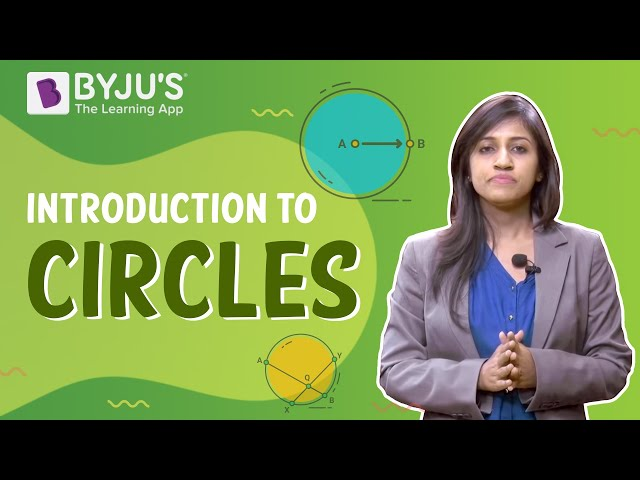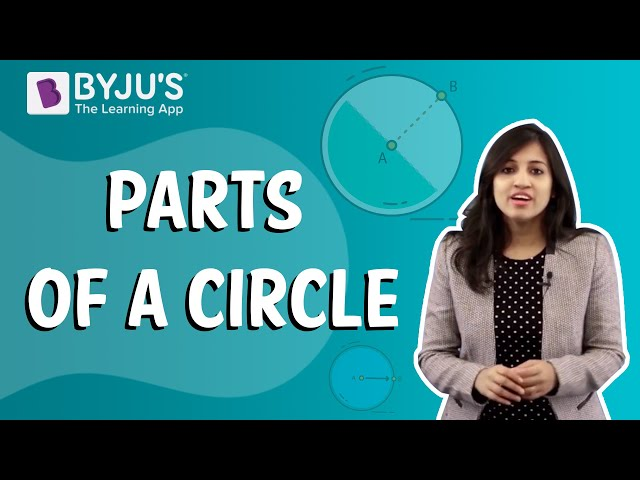# Problems On Area And Circumference Of A Circle

Before looking at problems on a circle based on perimeter and area, we need to understand the meaning of a circle and both the properties of the circle. In geometry, a circle is a closed two-dimensional figure in which the set of all the points in the plane is equidistant from a given point called the centre. Let’s have a look the definitions of perimeter and area given below:

## Perimeter Definition

Perimeter is associated with any closed figure like triangle, quadrilateral, polygons or circles. It is the measure of distance covered while going around the closed figure on its boundary.

For example, the perimeter of a square of side 2 cm = 8 cm, as we know that the square comprises 4 sides having equal lengths, thus the total distance covered will be 4×2, which will be the total length (i.e. perimeter).

## Area Definition

Area means the actual space enclosed by a closed figure (or within the perimeter), and it means all the points within the closed figure and not the boundary.

### Perimeter and Area of Circle Formula

Now, coming to the perimeter of a circle, as explained above, is the measure of distance going around the circle’s boundary. This distance is difficult to calculate precisely, and it is easy to visualize that the distance to go around is larger in a larger radius than a smaller radius. Hence, the perimeter is a function of the radius of a circle. In the case of the circle, we generally use the term “CIRCUMFERENCE” instead of the perimeter.

It is given by,

Circumference = 2πr

Here, r is a radius, and π is a constant defined as the ratio of circumference to the circle’s diameter.

The value of π is 22/7 or 3.1416

Also check: Circumference of a circle

The formula to find the area of a circle is given as:

A = πr2

### Solved Problems on Circle

Let us understand the concepts related to circles along with the following questions-

Example 1:

To cover a distance of 10 km, a wheel rotates 5000 times. Find the radius of the wheel.

Solution:

Number of rotations = 5000.

Total distance covered = 10 km

Let ‘r’ be the radius of the wheel.

Circumference of the wheel = Distance covered in 1 rotation = 2πr.

In 5000 rotations, the distance covered = 10 km = 1000000 cm.

$$\begin{array}{l}\text{Hence, in 1 rotation, the distance covered = }\frac{1000000}{5000}cm=200\: cm\end{array}$$

But this is equal to the circumference. Hence, 2πr = 200 cm

r = 200/2π

r = 100/π

Taking the approximate value of π as 22/7, we get

r = 100 x 7/22

r = 31.82 cm approx.

Example 2:

The diameter of a semicircular shape is 14 cm. What will be the perimeter of this shape?

Solution:

Given,

Diameter of semicircle = d = 14 cm

Radius = r = d/2 = 14/2 = 7 cm

Perimeter of semicircle = (Perimeter of circle/2) + d

= (2πr/2) + d

= πr + d

= (22/7) × 7 + 14

= 22 + 14

= 36 cm

Example 3:

The difference between the circumference and the diameter of a circular bangle is 5 cm. Find the radius of the bangle. (Take π = 22/7)

Solution: Let the radius of the bangle be ’r’

According to the question:

Circumference – Diameter=5 cm

We know, Circumference of a circle = 2πr

Diameter of a circle = 2r

Therefore, 2πr – 2r =5 cm

2r(π-1) = 5 cm

$$\begin{array}{l}2r(\frac{22}{7}-1)=5cm\\ \\ 2r\times \frac{15}{7}=5\\ \\ r=\frac{5\times 7}{15\times 2}\\ \\ r=1.166cm\end{array}$$

The radius of bangle is 1.166 cm.

Example 4:

A girl wants to make a square-shaped figure from a circular wire of radius 49 cm. Determine the sides of a square.

Solution: Let the radius of the circle be ’r’.

Length of the wire=circumference of the circle= 2πr

$$\begin{array}{l}= 2\times \frac{22}{7}\times 49=2\times 22\times 7\\ \\ =308\: cm\end{array}$$

Let the side of the square be ‘s’.

Perimeter of the square = length of the wire = 4s

$$\begin{array}{l}s=\frac{308}{4}\\ \\ s=77\:cm\end{array}$$

Therefore, the sides of the square is 77 cm.

Example 5:

Find the area of a circular region whose radius is 21 m.

Solution:

Given,

Radius of circular region = r = 21 m

Area of a circle = πr2

= (22/7) × 21 × 21

= 22 × 3 × 21

= 1386 sq. m

Therefore, the area of the circular region is 1386 sq. m.

## Video Lessons on Circles

### Introduction to Circles### Parts of a Circle### Area of a Circle